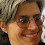## 2014-03-10

### Faulty Factoring

Here's something I think I see a few times in any college algebra class: a really weird way of accomplishing quadratic factoring. (More generally, this might go in a larger file of "things students swear are taught by other instructors which are semi-insane" -- including whacked-out order-of-operations, keep-change-change for negatives, the idea that -42 means (-4)(4), etc.).

Anyway, let's say we want to factor what I call a "hard quadratic", i.e., Ax2+Bx+C, in integers, with A≠1 (hence "hard"). I prefer the method of grouping: i.e., factor AC so it sums to B, use those factors to split the term Bx, and then factor the four terms by grouping. Pretty straightforward.

But here's what a few students will insist on doing every semester: (1) Find factors of AC that sum to B; call these factors u & v (so that step is the same); (2) Write the expression (Ax+u)(Ax+v); (3) Look for a GCF of A in one of those binomials and strike it out.

Here's an example: Factor 5x2+7x−6.
Step (1): Note AC = −30 = (10)(−3), factors which sum to B = 7.
Step (2): Write (5x+10)(5x−3)
Step (3): Divide the first binomial by 5, producing (x+2)(5x−3).

So while this procedure does produce the right answer, what irks me tremendously is that the expression written in step (2) is not actually equal to either the original expression or the answer at the end. (Compounding this issue, students will nonetheless usually write equals signs by rote on either side of it.) Riffs on this procedure would be to write something like this on sequential lines, if you can follow it:

5x2+7x−6 → x2+7x−30 → (5x+10/5)(5x−3/5) → (x+2)(5x−3)

Again, the primary grief I have over this is that none of these expressions are equal to any of the others, and the students using this procedure are always oblivious to that fact. Second issue: They're likely to trip up over a non-elementary problem where the factor A does not appear in either of the binomials, e.g.: 4x2+4x+1 = (2x+1)(2x+1). Third issue: If there's a GCF in the quadratic itself and you overlook that, the standard grouping technique will still work (even if it's not the easiest way to do it), whereas I suspect users of this technique will be prone to incorrectly striking out any GCFs they discover at the end of the process.

Now, technically you could modify this and turn it into a correct procedure this way: Note that for quadratic Ax2+Bx+C, values u & v satisfy uv=AC and u+v=B if and only if Ax2+Bx+C = 1/A(Ax+u)(Ax+v). (Proof: 1/A(Ax+u)(Ax+v) = 1/A(A2x2 + (u+v)Ax + uv) = Ax2 + (u+v)x + uv/A and equate coefficients). So you could find u & v as usual, then write this latter expression, and simplify. The 1/A does always cancel out, but I've never seen a student actually write that factor in the second step.

So what I always do if I see this on a test in my college algebra class is to take half credit off for the problem and note that the intermediary expression is "false", i.e., not equal to what comes before or after. This then becomes an opportunity to discuss with the student why that's improperly written math -- went well in my most recent semester, but I can easily see that becoming more combative in a remedial algebra class.

Have you seen this (common) faulty factoring procedure in your classes? What do you as a correction for it, if anything?

1.The first time I saw it, I was blown away. (And it isn't common among my students (CA now, MI previously).) I asked the student to explain what she was doing, and of course she couldn't. I couldn't see why it worked at first. I finally got her to show me enough examples that I figured out the real process, as you show above.

I let her use it. (Beginning algebra course, not worth the agony of pushing her to do something else, and she wasn't going to really understand it. Maybe now, 10 years later, I'd choose differently.)

1.Fascinating! At least I'm not the only one who sees this. I get maybe one or two students out of 30 doing this in those classes. I do have to keep a "backup explanation" prepared to engage with students when this becomes an issue.

2.I've seen that approach used before and have similar concerns with students getting problems wrong for the reasons you've noted.

A few years ago, I came up with an alternate approach to factoring quadratics that is a decomposition of the box method used to teach FOIL in Algebra 1. It turns out that it is not an original approach--others in history discovered it before me--but it is not commonly taught, or known by most math teachers, because it doesn't show up in most standard high school textbooks.

If anyone is curious, you can view a power point of the method at the link below (the second file). Note: when factoring out the GCF in slide 5, you always pull out the sign of the x coefficient, i.e. the GCF of -6x and 8 (for the method to work) is -2.

Glenn Laniewski
Blog:
autismplusmath
My method:
Factoring Quadratics by the Box Method

1.Well, that does work, and I'd say it's equivalent to the standard "factor by grouping" method (including the need to factors negatives with the GCF) -- except that using purely the GCFs from the box leaves it kind of a mystery as to why it works (and loses one place that one can double check). For example, I'd see your problem done as:

9x^2-18x+8
= 9x^2-12x-6x+8
= 3x(3x-4)-2(3x-4)
= (3x-4)(3x-2)

So: (1) that's what I see in any algebra textbook, (2) it allows a clear explanation of why the last step works (the common binomial counts as a GCF), and (3) there's an opportunity to double-check if the parenthetical binomials don't match.